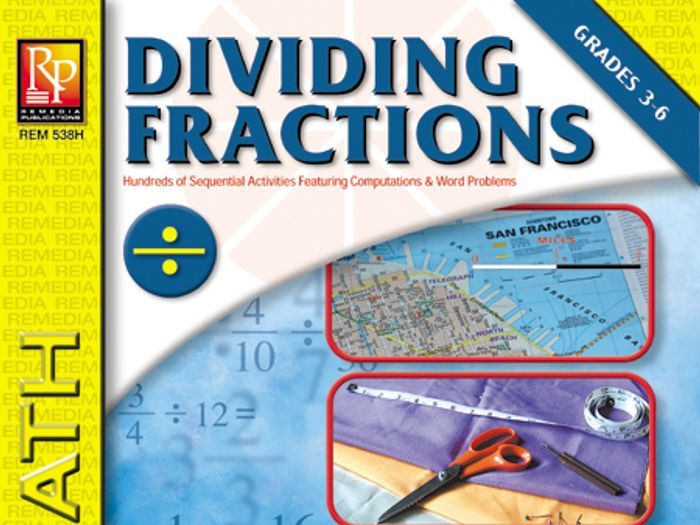Breaking down fractions!

This 25-lesson learning unit is packed with hundreds of sequential fraction activities featuring both computation and word problems. As the fourth book in a series – following Adding Fractions, Subtracting Fractions, and Multiplying Fractions – these exercises are designed to build upon what students have already learned.

From “dividing proper fractions by a whole number” to “solving story problems with the division and multiplication of fractions,” the exercises in this book progress from learning basic concepts, to mastering an understanding of how to divide fractions, and on to practicing both multiplying and subtracting fractions.

A Post Test and Answer Key are included.

£3.16

### Info

Created: May 5, 2017

Updated: Feb 22, 2018

pdf, 447 KB

EREM-538Hs

Report a problem

### Tes Paid Licence

How can I re-use this?